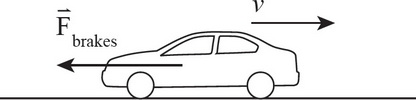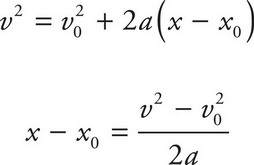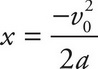# AP Physics 1 Question 381: Answer and Explanation

### Test Information

Question: 3819. A car travelling at a speed of v0 applies its brakes, skidding to a stop over a distance of x m. Assuming that the deceleration due to the brakes is constant, what would be the skidding distance of the same car if the braking were twice as effective (doubling the magnitude of deceleration)?

• A. 0.25x m
• B. 0.5x m
• C. x m
• D. 2x m

Explanation:

B

The variables involved in this question are the initial velocity (given in both cases), the acceleration (constant), final velocity (0 in both cases), and displacement (the skidding distance). As the missing variable is time, use Big Five #5 to solve for the displacement:As the initial position, x0, and the final velocity, v2, are equal to 0, this equation simplifies toWhen the acceleration is doubled, the final position is halved. (Note that the acceleration in this problem is negative, as the brakes cause the car to decelerate.)